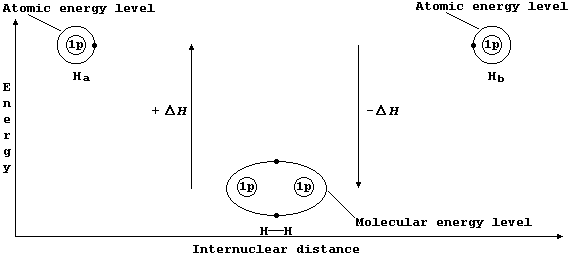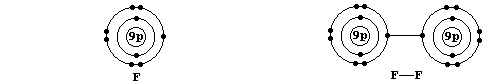```SELECTED PRINCIPLES: INTRODUCTION - LOCALIZED COVALENT BONDING (1)
Bonding between atoms, which occurs because the resulting molecule or
compound has a lower energy than its constituent atoms, is achieved by
redistributing the valence (or bonding) electrons. In covalent bonding,
this redistribution occurs by the atoms sharing two or more electrons.
The term localized covalent bond describes the mutual electrostatic
attraction of two adjacent nuclei for a shared pair of electrons which
occupy the same molecular energy level.
The Mechanism of Covalent Bonding
Consider two hydrogen atoms, Ha and Hb. The (potential) energy of each
electron, in its own separate atomic energy level, is reduced when both
electrons enter a molecular energy level which encompasses both nuclei;
in doing so, and forming a localized covalent bond, potential energy is
transduced to heat energy. So, bond formation is an exothermic process:
Ha(g) + Hb(g) ——————————® H——H(g)  DH = -432 kJ mol-¹  *``````Localized Single Bonds
The sketch graph above includes a method of representing the electronic
structure of dihydrogen which is a fairly reasonable approximation to
physical reality; in particular, it emphasizes that - after bonding -
the two electrons are indistinguishable from one another and that they
occupy one molecular energy level (not separate atomic energy levels).
However, similar representations for more complex molecules are rarely
used because the resulting diagrams are inevitably more complicated.
Shown below, for dihydrogen, is the simplest type of electron-structure
diagram.``````The merits of this type of electron-structure diagram are two-fold.
First, the retention of the atomic energy levels simplifies 'electronic
book-keeping'. And second, there is a clear and direct relationship to
one standard method of representing a molecule: i.e., by its structural
formula.
*  Conversely, bond breaking, as in the dissociation of dihydrogen, is
an endothermic process;
H——H(g) ——————————® Ha(g) + Hb(g)  DH = +432 kJ mol-¹```

```Shown below are electron-structure diagrams for atomic fluorine and
molecular fluorine (or difluorine).``````A fluorine atom contains seven electrons in its outermost atomic energy
level; because one of these is unpaired, such an atom is known as a
free-radical. The potential energy of this unpaired electron is reduced
when it becomes part of a covalent bond; as occurs, for example, when a
molecule of difluorine is formed.
The covalent bond in difluorine is formed in the same manner as that in
dihydrogen. Thus, one electron from the outermost atomic energy level
of each fluorine atom enters a molecular energy level which encompasses
both nuclei; the other (non-bonding) electrons form lone-pairs.
Difluorine is an example of a molecule which conforms to the so-called
'octet rule'; i.e., an atom - other than H, He, Li, Be, or B - tends to
form bonds until it is surrounded by eight valence electrons.  [This is
a useful rule of thumb for the electronic structures of molecules which
contain only carbon, nitrogen, oxygen, or fluorine. Nevertheless, there
are innumerable examples of compounds which contain bonded atoms that
have either an 'incomplete' or an 'expanded' octet.] *
Shown below are electron-structure diagrams for hydrogen fluoride and
water.``````Because the bonds in these two molecules are formed in the same manner,
and for the same reason, as both dihydrogen and difluorine, one might
expect no further comment. But, together, these four molecules beg an
important question: are all covalent bonds the same? The short answer
is no! However, in an introductory text, quite how much detail should
accompany this flat answer is difficult to judge; and so, rather than
throwing caution to the winds, only three brief points are made here.
First, by analogy with the different energy levels observed in atoms,
one would expect there to be different molecular energy levels. Indeed,
although well beyond the scope of this text, both spectroscopic studies
and theoretical calculations provide support for such an expectation;
e.g., the molecular energy levels associated with the covalent bonds in
H-H, F-F, H-F, and H-O-H are all different.
Second, perhaps intuitively, one might expect that the electrons are
not equally distributed when the two nuclei are different; the unequal
distribution of 'electron density' is known as polarization (and occurs
in both H-F and H-O-H, for example: but not in either H-H or F-F).
And third, quantitative determinations of molecular energy levels and
polarization, amongst other bonding parameters, allow many reactions of
molecules to be both correlated and predicted.
*  As a caveat to the 'octet rule', consider the following. Each of the
46 chromosomes present in (most) cells of the human body contain bonded
phosphorus which does not adopt the electronic structure of argon. [...
And setting aside identical sprogs, the exponentially increasing human
population is?  ... And setting aside the depressing fact that numbers
are ever decreasing, because of pollution, the number of species is?]
Dr. R. Peters                   Next                     Contents' List```